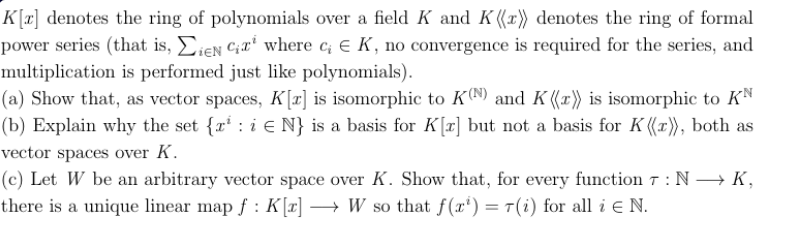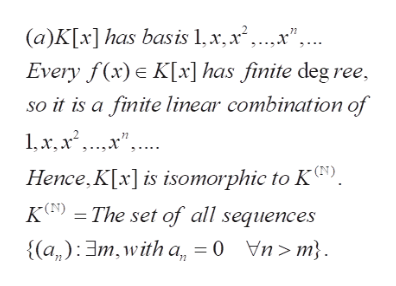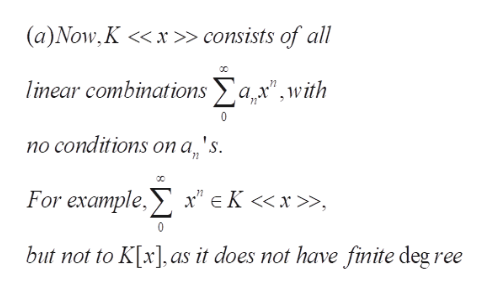K[T denotes te ring of polynomials over a field K and K ((x) denotes the ring of formalpower series (that is, ieN Cwhere c E K, no convergence is required for the series, andmultiplication is performed just like polynomials)(a) Show that, as vector spaces, Kz] is isomorphic to K0N9) and K (x') is isomorphic to KN|(b) Explain why the set {r i E N} is a basis for Kr] but not a basis for K ((r), both asvector spaces over K(c) Let W be an arbitrary vector space over K. Show that, for every function 7: N Kthere is a unique linear map f: K [a] -> W so that f(x') = T(i) for all i E N.

Question

Please, help me with a very detailed and self-explanatory solution to this problem. I will appreciate it alot. Thank youhelp_outlineImage TranscriptioncloseK[T denotes te ring of polynomials over a field K and K ((x) denotes the ring of formal power series (that is, ieN Cwhere c E K, no convergence is required for the series, and multiplication is performed just like polynomials) (a) Show that, as vector spaces, Kz] is isomorphic to K0N9) and K (x') is isomorphic to KN |(b) Explain why the set {r i E N} is a basis for Kr] but not a basis for K ((r), both as vector spaces over K (c) Let W be an arbitrary vector space over K. Show that, for every function 7: N K there is a unique linear map f: K [a] -> W so that f(x') = T(i) for all i E N. fullscreen
Step 1

Problem concerns the ring and vector space structure of the space of formal power series over K, K a field

Step 2

(a) Here K[x] is the ring of polynomials over K ; note that any polynomial consisists only finitely many terms . Thus , as a vector space ,K[x] is isomorphic to the space of sequences over K which are eventually 0, (as described in the last two lineshelp_outlineImage Transcriptionclose(a)K[x] has basis 1,x, x ,...x", Every f(x)e K[x] has finite deg ree, so it is a finite linear combination of Hence.K[x]is isomorphic to K). K () The set of all sequences {(a,):m, with a, 0 fullscreen
Step 3

(a) in contrast, K<<x>> is the ring of formal power series , here an element of K<<x>> can have infinitely many non-zero coefficients....help_outlineImage Transcriptionclose(a)Now, K << x» consists of all linear combinationsax",with no conditions on a 's 71 For example, x"eK <» but not to K[x], as it does not have finite deg ree fullscreen

Want to see the full answer?

See Solution

Want to see this answer and more?

Our solutions are written by experts, many with advanced degrees, and available 24/7

See Solution
Tagged in

Math